MCAT Physical : Gases

Example Questions

← Previous 1 3 4

Example Question #1 : Using Molar Volume

What is the volume of two moles of diatomic nitrogen gas at STP?

22.4L

33.6L

11.2L

44.8L

44.8L

Explanation:

One mole of gas has a volume of 22.4L at STP.

Since there are two moles of gas present, the volume is doubled.

2(22.4) = 44.8L

The fact that the gas is diatomic is irrelevant.

Example Question #1 : Using Molar Volume

Air is composed of about 78% nitrogen (molecular mass = 28 g/mole), 21% oxygen (molecular mass = 32 g/mole), and 1% argon (40 g/mole). The weighted average of these numbers leads to the approximation that if air was a pure gas, its “molar mass” would be about 29 g/mole. Helium has a molar mass of 4 g/mole.  What is the volume of helium gas required to lift a balloon carrying a 100 gram sensor?

3

2

1

5

4

3

Explanation:

Choice 3 is correct. The question forces the respondent to recall that one mole of an ideal gas occupies 22.4 liters at standard temperature (zero Celsius) and pressure (1 atmosphere). Helium balloons rise because they are less dense than air by a factor of 29 g/mole – 4 g/mole = 25 g/mole. Since the sensor has a mass of 100 g, it will require about 4 molar volumes of He to lift it.

Example Question #1 : Gas Laws

15L of a gas is held at constant pressure as the temperature increases from 300K to 350K. What is the new volume?

17.5L

The volume does not change

10L

12.5L

17.5L

Explanation:

Charles's Law states that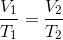. To solve for the final volume, we simply plug in our given values to this equation.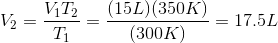This law applies only for isobaric (constant pressure) changes.

Example Question #1 : Gas Laws

8 liters of an ideal gas is in an isolated container at 30 degrees Celsius. The container is heated at constant pressure until its volume is doubled. What is the new temperature of the gas?

150oC

333oC

220oC

124oC

60oC

333oC

Explanation:

At constant pressure,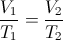, where the temperatures are measured in Kelvin (absolute temperature).

First, convert the given temperature (C) to Kelvin (K).

K = C + 273 = 30 + 273 = 303K

Plug the temperature and volumes into the above equation and solve for the final temperature.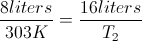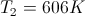Convert this value back to Celsius.

C = K - 273 = 606 - 273 = 333oC

Example Question #3 : Gas Laws

Five moles of nitrogen gas are present in a 10L container at 30oC. What is the pressure of the container?

9atm

5atm

10.5atm

12.4atm

17.2atm

12.4atm

Explanation:

Using the ideal gas law equation we can find that P= nRT/V. We then plug in the given values.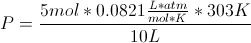Solving for P gives us 12.4atm.

Note: 30oC must be converted into Kelvin by adding 273K

Example Question #4 : Gas Laws

If one mole of oxygen gas occupies a 5L container at 300K, what is the pressure in the container?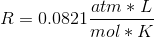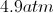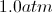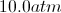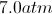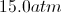Explanation:

Using the ideal gas law,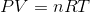, we can solve for pressure.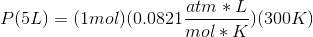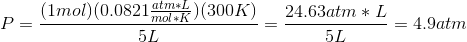Example Question #5 : Gas Laws

Recall that the ideal gas law states that, where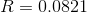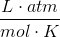.

If there are 5g of hydrogen gas in a 10L chamber at 32°C, what would be the pressure?

6.25atm

3.15atm

12.5atm

1.3atm

6.25atm

Explanation:

Using the equationand solving for P you get,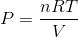.

Recall that hydrogen forms a diatomic molecule when in gas form. This should always be an assumption when working with hydrogen gas on the MCAT. When we convert 5g to moles, we must use a conversion factor of 2g/mol.Temperature must be converted to Kelvin. You must have this conversion memorized for the MCAT.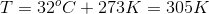Now we can solve for P.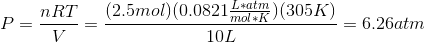Example Question #6 : Gas Laws

A certain gas is initially at a pressure of 2atm in a volume of 5L. It then experiences a decrease in volume to 2L, and is held at a constant temperature throughout the process. What is the new pressure?

2atm

3atm

5atm

4atm

5atm

Explanation:

Since this is an isothermal change (constant temperature), this falls under Boyle's law.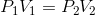Taking this equation and solving for the new pressure (P2) we come up with 5atm.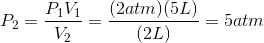Example Question #7 : Gas Laws

A balloon filled with one mole of an ideal gas is leaking molecules at a constant rate of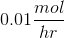.

After 75 hours, the pressure is half of the initial pressure. What is the new volume in terms of the initial volume,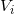?

Assume temperature remains constant.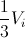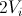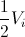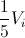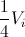Explanation:

We can use the given values to determine how many moles of the gas have leaked out after 75 hours.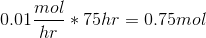We now know that the balloon started with one mole, and that 0.75 moles have leaked. This means that 0.25 moles remain in the balloon. We now have our initial and final mole values and pressure values. We can rearrange the ideal gas law to isolate the variables we need, assuming that the temperature is constant.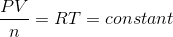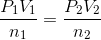Using our proportions, we can try to solve for the final volume. For simplicity, assume the initial pressure is 1 and the final pressure is 0.5.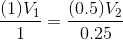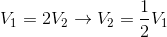Example Question #8 : Gas Laws

How many moles of oxygen gas are in a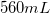sample that is at a temperature of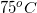and a pressure of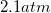?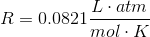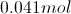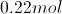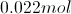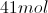Explanation:

First, each value must be converted to the correct units given for the gas constant.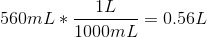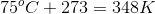Next, use the ideal gas law to solve for moles.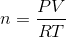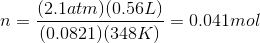← Previous 1 3 4